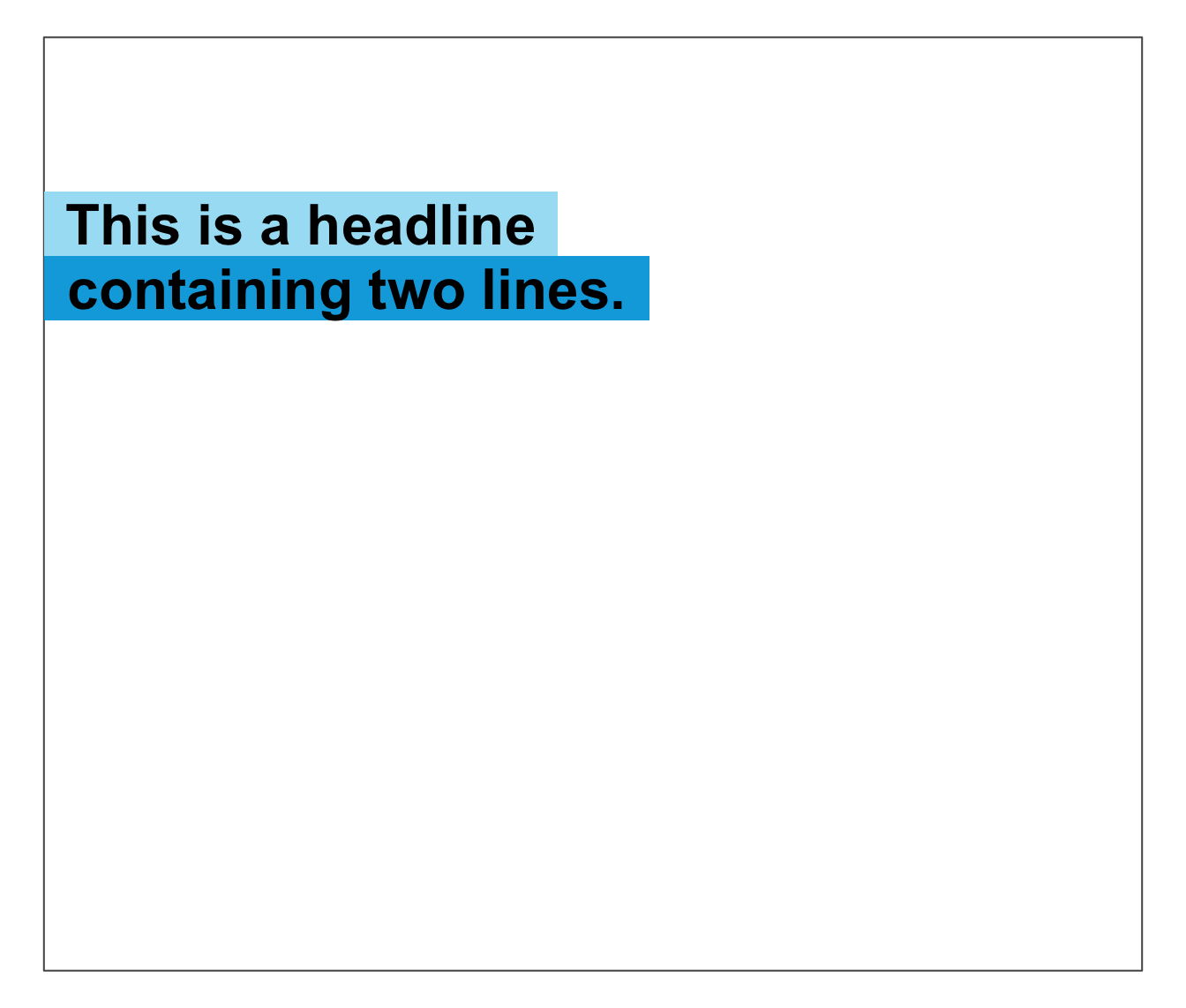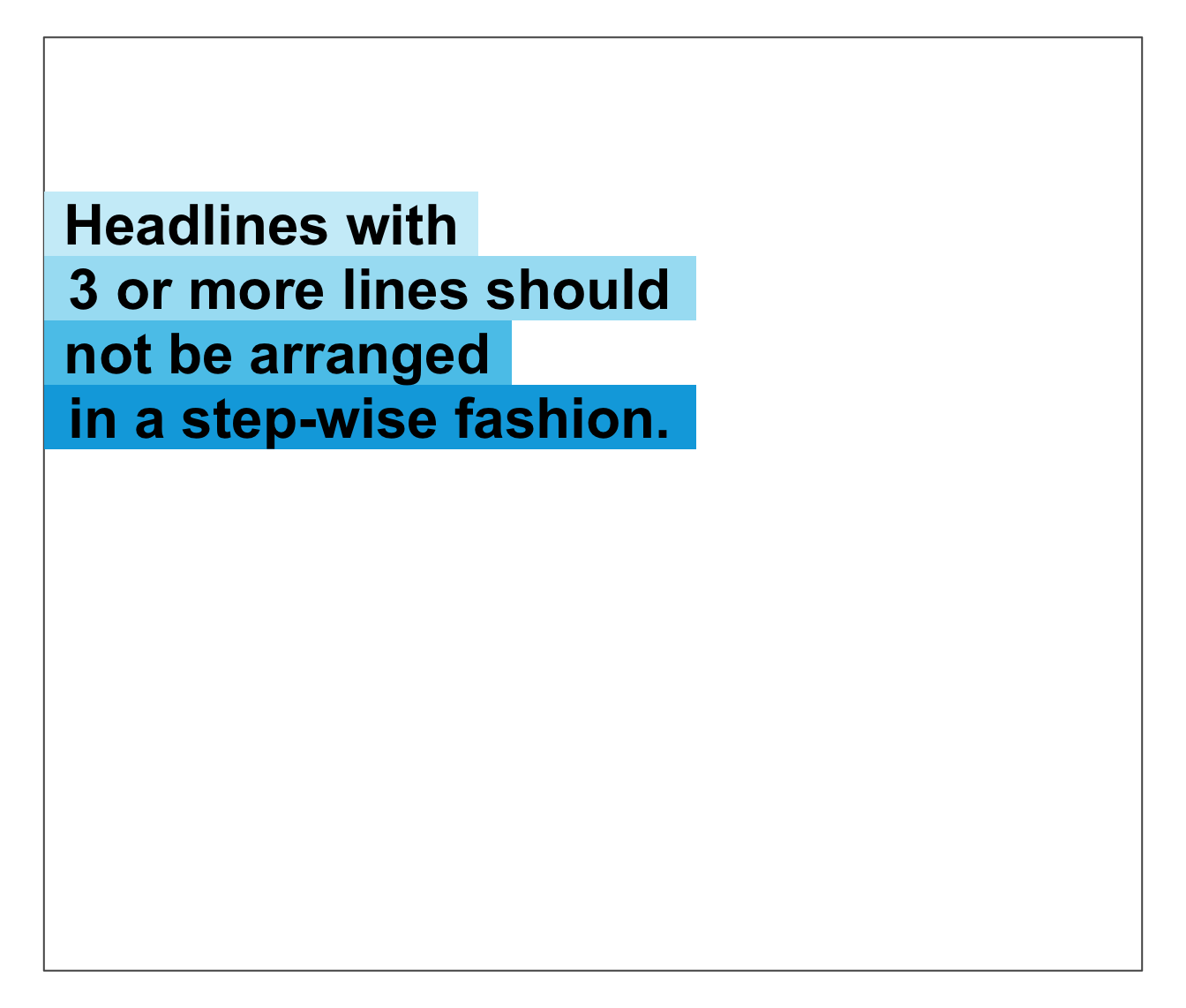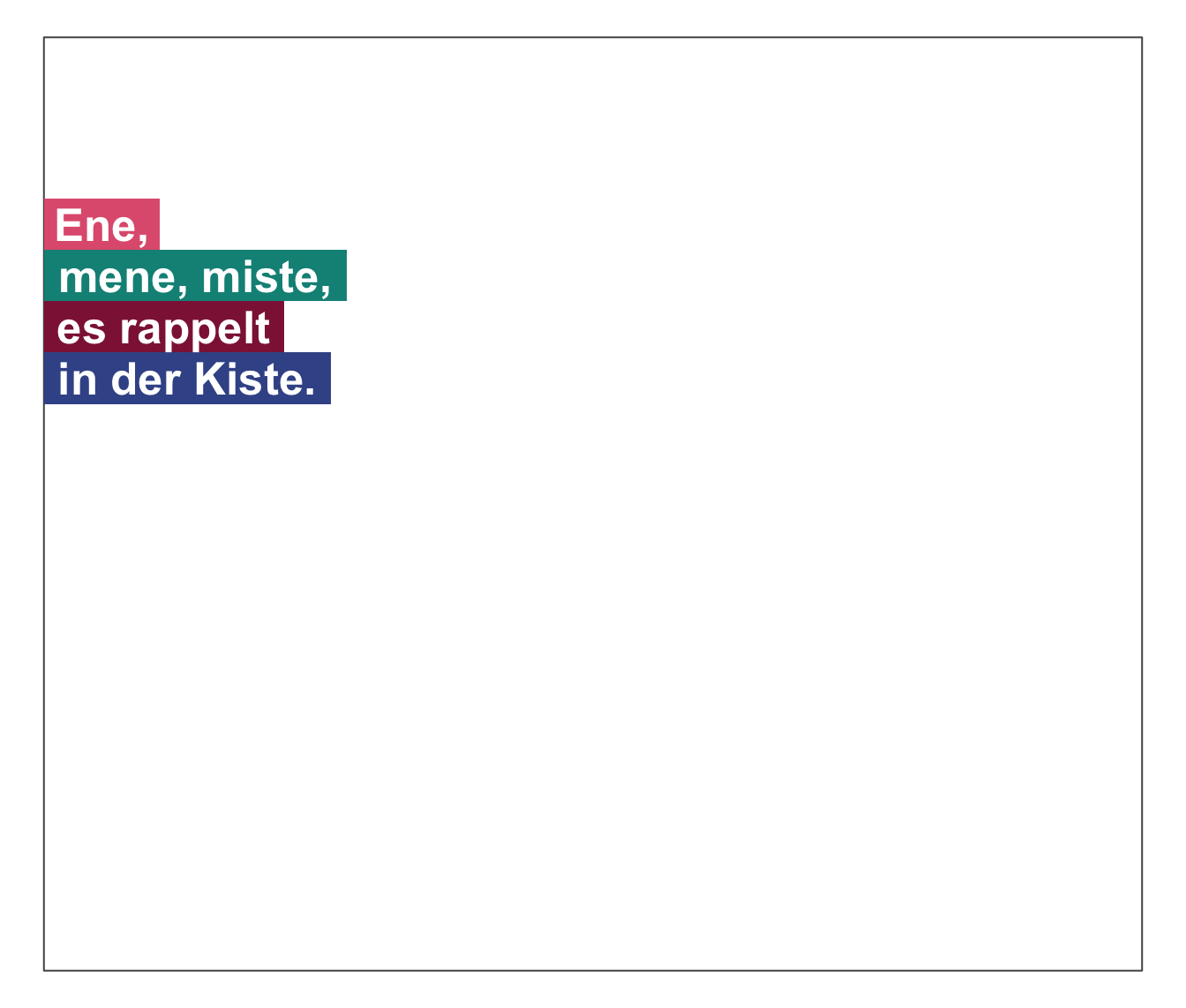heading plots 1 or more text strings (provided as a character vector labels) as a heading to an (existing or new) plot and places a colored box behind each label to mark it (i.e., highlighting the heading).

labels,
x = 0,
y = 0.8,
y_layout = "flush",
col = "black",
col_bg = "default",
cex = 2,
font = 2,
new_plot = "slide"
)

Arguments

labels A character vector specifying the text labels to be plotted. A numeric vector of x-coordinates at which the text labels in labels should be written. If the lengths of x and y differ, the shorter one is recycled. Default: x = 0. A numeric vector of y-coordinates at which the text labels in labels should be written. If the lengths of x and y differ, the shorter one is recycled. Default: y = .8. A numeric value or vector for the vertical spacing of labels in labels. 2 special values are "even" (i.e., even distribution of labels across available y-space) and "flush" (i.e., no space between adjacent labels, or y_layout = 0). Default: y_layout = "flush". The color(s) of the text label(s). Default: col_lbl = "black". The color(s) to highlight or fill the rectangle(s) with. Default: col_bg = "default" (to automatically select different shades of pal_seeblau). Numeric character expansion factor(s), multiplied by par("cex") to yield the character size(s). Default: cex = 2. The font type(s) to be used. Default: font = 2 (i.e., bold). Boolean: Should a new plot be generated? Set to "blank" or "slide" to create a new plot, and to "none" to add to an existing plot. Default: new_plot = "slide" (i.e., create a new slide).

Details

Text formatting parameters (like col, col_bg, cex, font) are recycled to match length(labels).

heading uses the base graphics system graphics::.

slide and xbox to create simple plots (without text).

Examples